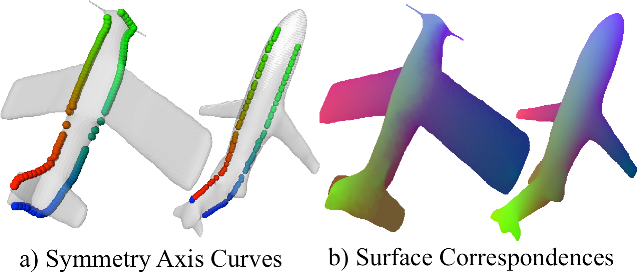PrincetonComputer SciencePIXL GroupPublications → [Liu et al. 2012]
Finding Surface Correspondences Using Symmetry Axis Curves

Computer Graphics Forum (Proc. Symposium on Geometry Processing), July 2012

Tianqiang Liu, Vladimir G. Kim, Thomas FunkhouserAligning symmetry axis curves helps find surface correspondences. Given two 3D models, we extract symmetry axis curves from both, align the curves (left), and then extrapolate the axis correspondences to produce a full surface map (right). In these images, points with the same color are predicted correspondences.
Abstract

In this paper, we propose an automatic algorithm for finding a correspondence map between two 3D surfaces. The key insight is that global reflective symmetry axes are stable, recognizable, semantic features of most real-world surfaces. Thus, it is possible to find a useful map between two surfaces by first extracting symmetry axis curves, aligning the extracted curves, and then extrapolating correspondences found on the curves to both surfaces. The main advantages of this approach are efficiency and robustness: the difficult problem of finding a surface map is reduced to three significantly easier problems: symmetry detection, curve alignment, and correspondence extrapolation, each of which has a robust, polynomial-time solution (e.g., optimal alignment of 1D curves is possible with dynamic programming). We investigate of this approach on a wide range of examples, including both intrinsically symmetric surfaces and polygon soups, and find that it is superior to previous methods in cases where two surfaces have different overall shapes but similar reflective symmetry axes, a common case in computer graphics.
Paper
Citation

Tianqiang Liu, Vladimir G. Kim, and Thomas Funkhouser.
"Finding Surface Correspondences Using Symmetry Axis Curves."
Computer Graphics Forum (Proc. Symposium on Geometry Processing), July 2012.

BibTeX

```@article{Liu:2012:FSC,
author = "Tianqiang Liu and Vladimir G. Kim and Thomas Funkhouser",
title = "Finding Surface Correspondences Using Symmetry Axis Curves",
journal = "Computer Graphics Forum (Proc. Symposium on Geometry Processing)",
year = "2012",
month = jul
}
```